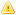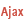Home |Sign in | EnglishHelpWLanguageWLanguage functionsControls, pages and windowsDrawing functionsPrefix syntaxDrawing functions on picLayer variablesDrawPolygonPresentationExampleCoordinatesDrawing with opacity or anti-aliasingPattern and PHPDrawing in Browser codeRelated examplesSee alsoAlphaBlendBackgroundChangeModeDrawChordDrawLineDrawPointDrawPolygonDrawPolylineDrawRectangleDrawRectangleGradientDrawRoundedRectangleDrawSliceDrawTextDrawTextRTFEndDrawingFillFontHorizontalSymmetryInvertColorPenPixelColorPixelOpacityResizeRotationStartDrawingVerticalSymmetryThis content has been translated automatically. Click here to view the French version.
• Coordinates
• Drawing with opacity or anti-aliasing
• Pattern and PHP
• Drawing in Browser code
WINDEVWEBDEVWINDEV MobileOthers<picLayer variable>.DrawPolygon (Function)
In french: <Variable picCalque>.DessinePolygone
Draws a polygon in an picLayer variable.Versions 15 and laterThis function is now available for WEBDEV sites in Linux.New in version 15This function is now available for WEBDEV sites in Linux.This function is now available for WEBDEV sites in Linux.
Example
MyWDPicImage is WDPic = "Test.gif"
MyLayer is picLayer

// Draw a 4-side polygon whose
// background is light yellow and whose border is light blue
MyLayer.DrawPolygon(4, 10, 20, 20, 50, 40, 60, 50, 10, LightYellow, LightBlue)
IMG_MyDrawing = MyWDPicImage
Syntax

Building a polygon point by point

<picLayer image>.DrawPolygon(<Number of sides> , <X1> , <Y1> , <X2> , <Y2> [, <X3> , <Y3> [... [, <Xn> , <Yn>]]] [, <Background color> [, <Side color>]])
<picLayer image>: picLayer variable
Name of the picLayer variable to use.
<Number of sides>: Integer
Number of sides in the polygon (up to 23 sides). This parameter defines the number of <X>, <Y> pairs to specify in the function.
<X1>: Integer
X coordinate of first point of the polygon. These coordinates are expressed in pixels.
<Y1>: Integer
Y coordinate of first point of the polygon. These coordinates are expressed in pixels.
<X2>: Integer
X coordinate of second point of the polygon. These coordinates are expressed in pixels.
<Y2>: Integer
Y coordinate of second point of the polygon. These coordinates are expressed in pixels.
<X3>: Integer
X coordinate of 3rd point of the polygon. These coordinates are expressed in pixels.
<Y3>: Integer
Y coordinate of 3rd point of the polygon. These coordinates are expressed in pixels.
<Xn>: Optional integer
X coordinate of nth point of the polygon. These coordinates are expressed in pixels.
<Yn>: Optional integer
Y coordinate of nth point of the polygon. These coordinates are expressed in pixels.
<Background color>: Integer or constant (optional)
Polygon background color. This color can correspond to:
If this parameter is not specified, the background color:
<Side color>: Integer or constant (optional)
Color of polygon sides. This color can correspond to:
If this parameter is not specified, the line color:
• corresponds to the Color specified in the last call to function <Image>.Pen,
• is identical to the Color used for the Background if the <Image>.Pen function has not been used.

Building a polygon via an array of X and Y coordinates

<picLayer image>.DrawPolygon(<Array of coordinates> [, <Background color> [, <Side color>]])
<picLayer image>: picLayer variable
Name of the Variable type picLayer to handle.
<Array of coordinates>: Array of integers or array of points
• WLanguage array of integers containing the coordinates of polygon points. The even subscript represent the X coordinates while the odd subscripts represent the Y coordinates of points. These coordinates are expressed in pixels.
•WLanguage array of Point variables containing the coordinates of polygon points. These coordinates are expressed in pixels.
<Background color>: Integer or constant (optional)
Polygon background color. This color can correspond to:
• an RGB color (returned by RGB),
• an HSL color (returned by HSL),
• une preset color of WLanguage,
•an Variable of the Color type.
If this parameter is not specified, the background color:
<Side color>: Integer or constant (optional)
Color of polygon sides. This color can correspond to:
• an RGB color (returned by RGB),
• an HSL color (returned by HSL),
• une preset color of WLanguage,
•an Variable of the Color type.
If this parameter is not specified, the line color:
• corresponds to the Color specified in the last call to function <Image>.Pen,
• is identical to the Color used for the Background if the <Image>.Pen function has not been used..Versions 26 and laterConstruction of a polygon from an Variable of the Polygon type

<picLayer image>.DrawPolygon(<Polygon> [, <Background color> [, <Side color>]])
<picLayer image>: picLayer variable
Name of the Variable type picLayer to handle.
<Polygon>: Variable type Polygon
Name of the variable of type Polygon describing the different points of the Polygon.
<Background color>: Integer or constant (optional)
Polygon background color. This color can correspond to:
If this parameter is not specified, the background color:
<Side color>: Integer or constant (optional)
Color of polygon sides. This color can correspond to:
If this parameter is not specified, the line color:
• corresponds to the color specified in the last call to <Image>.Pen,
• is identical to the Color used for the Background if the <Image>.Pen function has not been used..New in version 26Construction of a polygon from an Variable of the Polygon type

<picLayer image>.DrawPolygon(<Polygon> [, <Background color> [, <Side color>]])
<picLayer image>: picLayer variable
Name of the Variable type picLayer to handle.
<Polygon>: Variable type Polygon
Name of the variable of type Polygon describing the different points of the Polygon.
<Background color>: Integer or constant (optional)
Polygon background color. This color can correspond to:
If this parameter is not specified, the background color:
<Side color>: Integer or constant (optional)
Color of polygon sides. This color can correspond to:
If this parameter is not specified, the line color:
• corresponds to the color specified in the last call to <Image>.Pen,
• is identical to the Color used for the Background if the <Image>.Pen function has not been used..Construction of a polygon from an Variable of the Polygon type

<picLayer image>.DrawPolygon(<Polygon> [, <Background color> [, <Side color>]])
<picLayer image>: picLayer variable
Name of the Variable type picLayer to handle.
<Polygon>: Variable type Polygon
Name of the variable of type Polygon describing the different points of the Polygon.
<Background color>: Integer or constant (optional)
Polygon background color. This color can correspond to:
If this parameter is not specified, the background color:
<Side color>: Integer or constant (optional)
Color of polygon sides. This color can correspond to:
If this parameter is not specified, the line color:
• corresponds to the color specified in the last call to <Image>.Pen,
• is identical to the Color used for the Background if the <Image>.Pen function has not been used..
Remarks

Coordinates

Drawing a polygon:
MyImage is WDPic
MyLayer is picLayer

MyLayer.DrawPolygon(5, 80, 10, 10, 150, 100, 200, 160, 80, 140, 40)Coordinates are given with respect to the top left corner of the image (coordinates: (0.0)).
Important: There is no need to close the polygon (the coordinates of last point do not have to be equal to the coordinates of first point). The polygon is automatically "closed".
Limits: a polygon can contain up to 100 000 points in WINDEV, WEBDEV and Java, and up to 1000 points in Windows CE.Drawing with opacity or anti-aliasing

In the case of a drawing with opacity (function <picLayer variable>.StartDrawing with the constant dWithOpacity) or anti-aliasing (function <picLayer variable>.ChangeMode with the constant drawAntiAliasing), the contour is drawn over the inside of the Polygon: therefore, the line is partly combined with the inside of the polygon. If the line is very thick, it will be displayed in two colors.
Related Examples:Unit examples (WINDEV): The drawing functions [ + ] Using the main drawing functions of WINDEV to:- Initialize an Image control for drawing- Draw simple shapes- Write a text into a drawing- Change the color in a drawing
Business / UI classification: Neutral code
Component: wd260pnt.dll
Minimum version required
• Version 25